# Negative Exponents With Fractional Bases [Math]

in STEMGeeks3 months ago (edited)

Hi everyone. In this mathematics education post I cover the topic of negative exponents with fractional bases. It is assumed the reader is familiar with multiplying fractions, dividing fractions and some exponent properties.

Screenshots here are from witeboard.com with the use of my Wacom tablet & stylus.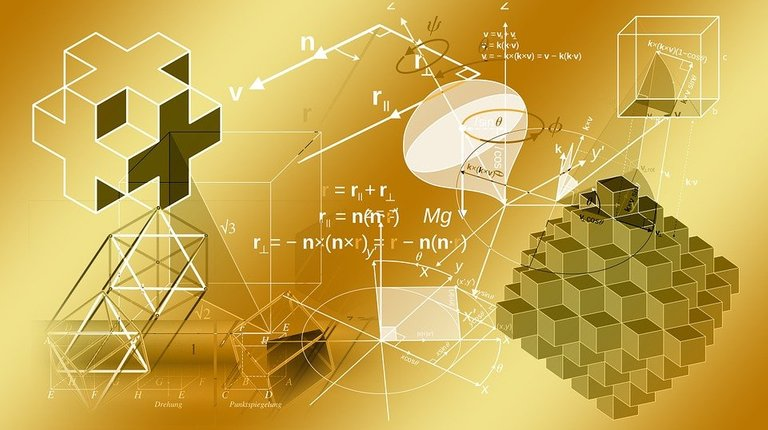Pixabay Image Source

## Topics

• Concept Of A Base & Exponent Review
• Fractional Bases
• Negative Exponents
• Combining The Two - Negative Exponents With Fractional Bases

## Concept Of A Base & Exponent Review

Representing something like `2 x 2 x 2 x 2 x 2 x 2` would take up a lot of space and would require a lot of writing/typing. A compact way of representing `2 x 2 x 2 x 2 x 2 x 2` would require the use of a base and an exponent. The number being multiplied is two so that would be the base. As the base of two is repeated six times the exponent here would be six.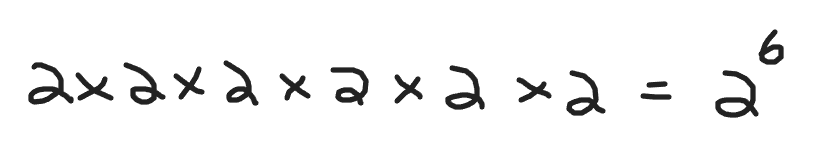Here is another example. Instead of having `10 x 10 x 10` we could do ten to the power of three. Ten is the base and three is the exponent.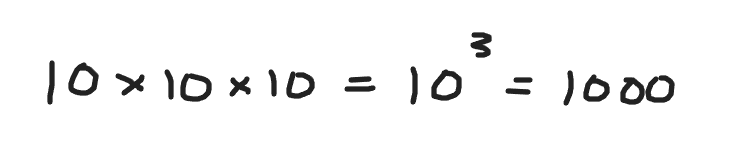We can generalize the base and exponent. Given a base of `x` and an exponent `n`. We have `x` to the power of `n`. For this post `n` is assumed to be a whole number positive or negative. Fractional exponents would be a different topic.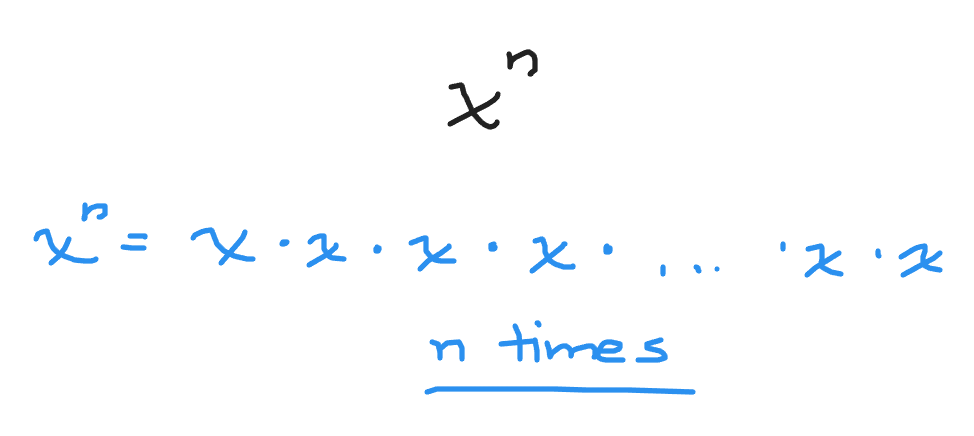## Fractional Bases

With fractional bases you have to be a little bit careful here. Here are some examples.

Example One

Half times half times half times half is equal to what number?

For learning purposes I write this half times half times half times half as repeated multiplication with the fraction of half.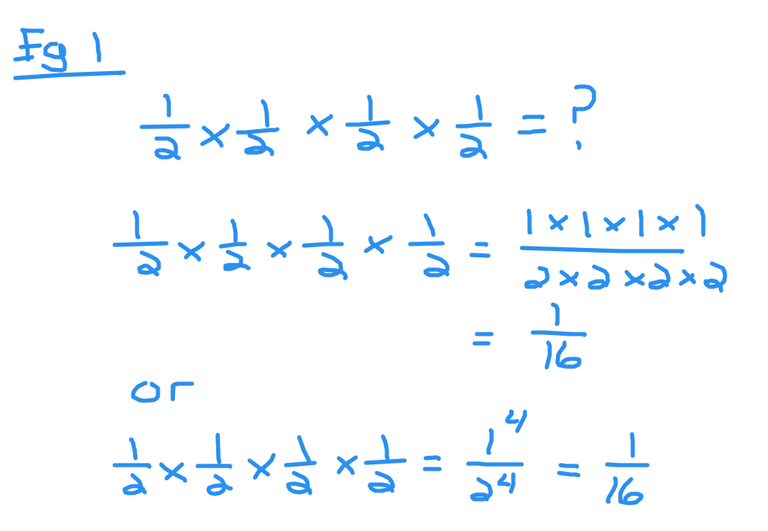This is a multiplying fractions cases. Multiply the ones on the top together to obtain one. Multiply the four twos on the bottom together to obtain sixteen.

An alternate solution would be one to to the power of four on the numerator (top of fraction) and two to the power of four (bottom of fraction).

Example Two

What is seven-thirds to the power of three?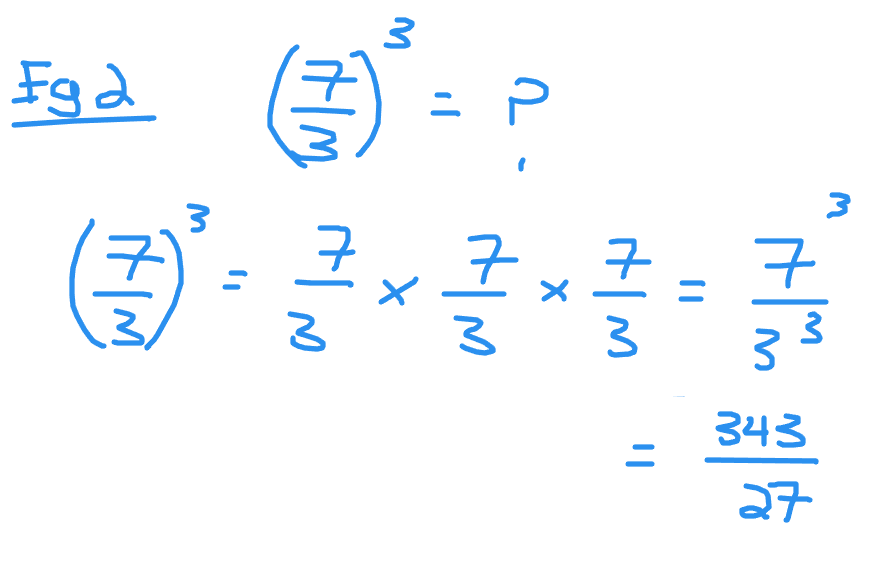You can rewrite seven thirds to the power of three as seven thirds times seven thirds times seven thirds. For the numerator it is seven to the power of three. With the denominator it is three to the power of three. The final simplified answer is 343 divided by 27.

Given a fractional base with an exponent we have the general formula/rule.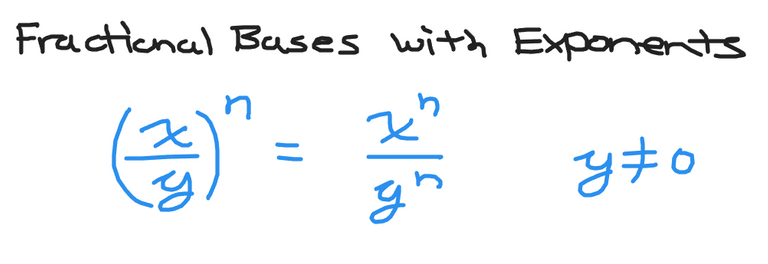## Negative Exponents

Negative exponents can be tricky. Do remember that the negative exponent means that we take the reciprocal of the base. Reciprocal means flip the fraction.

Here is the general formula for a whole number base with a negative exponent.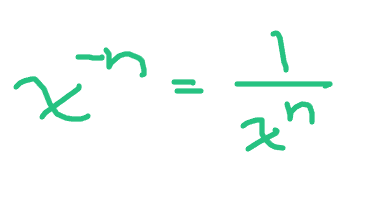Here is the case where the base is a fraction and the exponent is negative.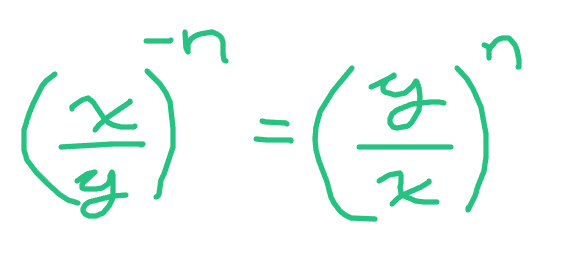One of the hardest cases is where you have the base and negative exponent on the denominator.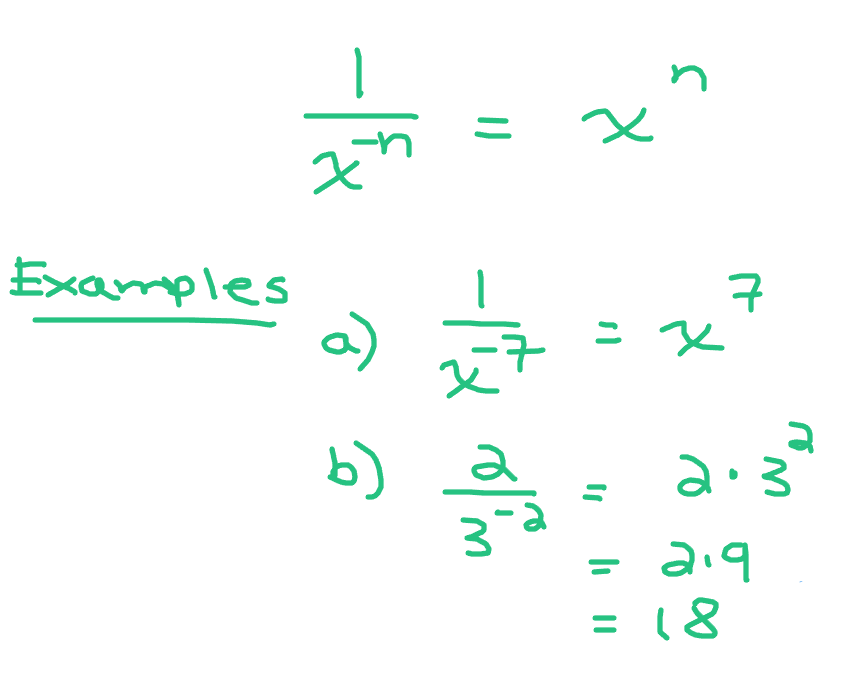Example One

What is three to power of negative two?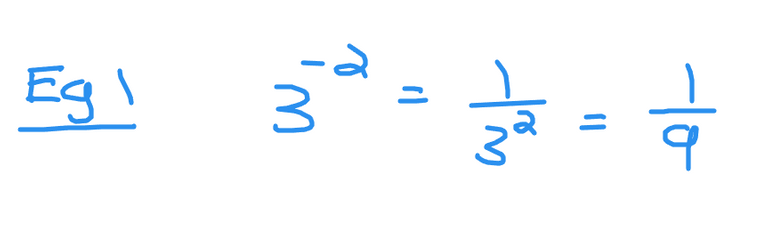Example Two

Determine the simplified value of two thirds to the power of negative four.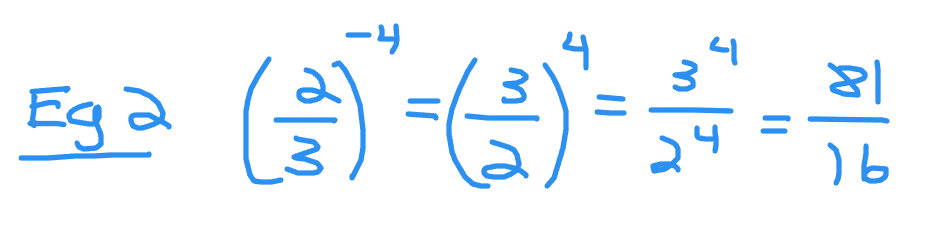&nbsp:

Example Three

What is five divided by `x` to the power of negative ten?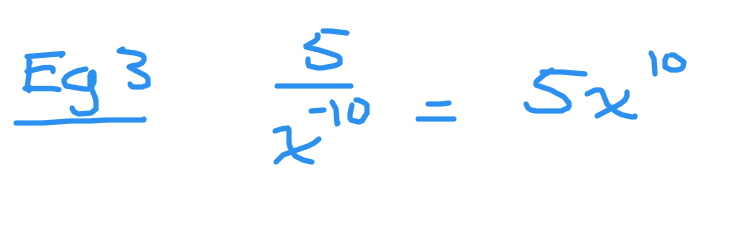## Combining The Two - Negative Exponents With Fractional Bases

Combining the two concepts of fractional bases and negative exponents leads to this formula.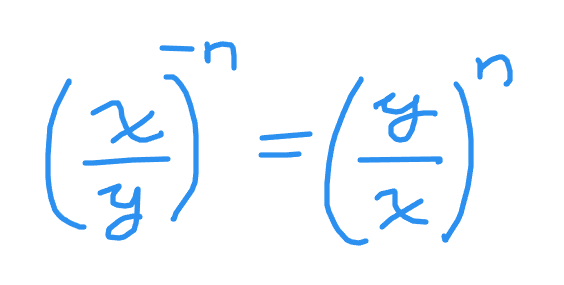Take the reciprocal of the fraction and convert the negative exponent into a positive exponent. In other words flip the fraction and change the sign of the exponent outside the bracket of the fraction.

Example One

Express the following with positive exponents. X-squared divided by `y` to the power of three with this fraction to the power of negative 10.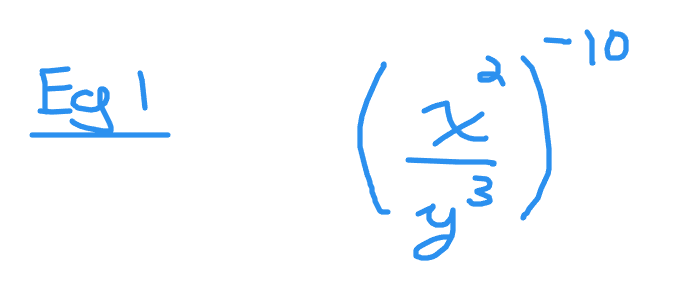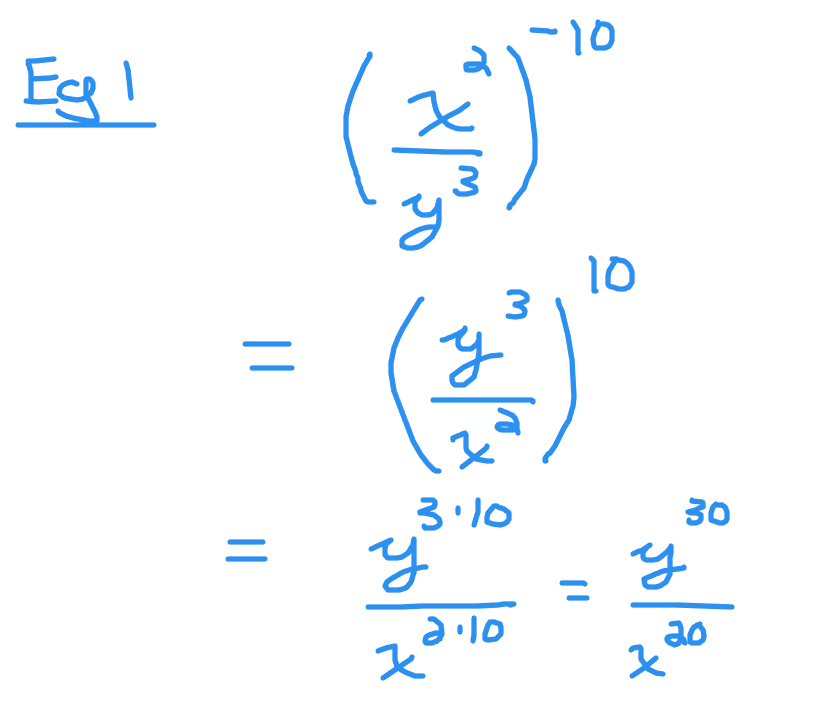Example Two

Simplify the following & convert into positive exponents.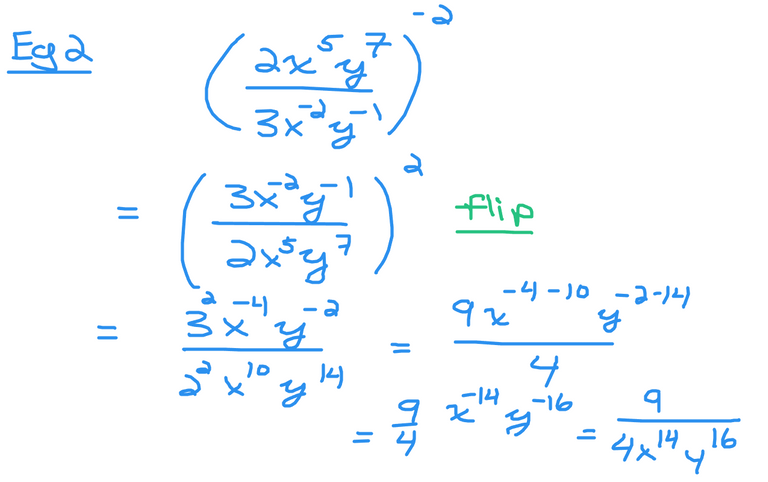Flip the fractions first and turn the negative two exponent into a positive two for the exponent for the fraction.

Distribute the exponent of two to the three, `x` to the power of negative two and the `y` to the negative one in the numerator of the fraction. Do the same with the denominator. That is distribute the two to the two, `x` to the power of five and `y` to the power of seven in the denominator.

Three squared is nine and two squared is four.

Apply exponent laws for the `x` variable and the `y` variable. Top exponent minus bottom exponent given that the base is the same.

To finish the negative exponent is the reciprocal with the positive exponent.

Example Three

Simplify the following fraction.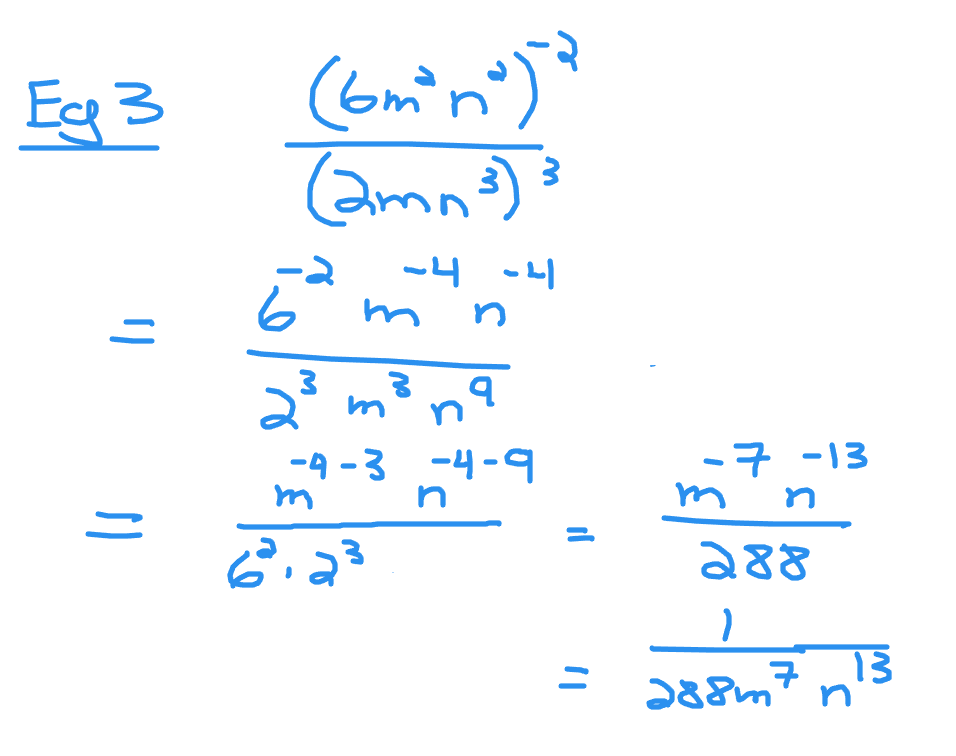In the numerator, multiply the negative two exponent with the six, m-squared and n-squared.

With the denominator, distribute the exponent of three to the two, m and n to the power of three.

Six to the power of two turns into 1 divided by 36. In this case the 36 moves to the bottom of the denominator.
Apply exponent laws for the m-variable and the n-variable.